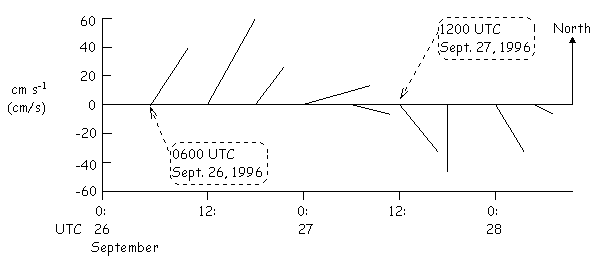How to Read a Stick Plot

After you've selected a buoy and clicked on the "View Image of Current Buoy Velocity Data" option to see a graph of the latest data, you are suddenly confronted with a page containing 4 graphs stacked on top of each other. The one you are interested in is the odd-looking one at the top. This graph is called a "stick plot" and the following are some tips on reading one of these graphs.

Each "stick" (also called a vector) on the stick plot contains three pieces of information: direction, time, and magnitude (strength).

Direction: It helps if you imagine a small arrowhead on the end of each stick (see the first stick in the example below). Then each arrow is pointing in the compass direction the current is going "to". The directions follow the conventions for hardcopy maps...a stick pointing to the upper right of the page is pointing northeast, a stick pointing to the bottom of the page is pointing due south, etc. (Side note: remember, wind is described by the direction it is coming "from", currents are described by the direction they are going "to".) The example below has the compass direction (NE = northeast, NNE = north northeast, etc.) posted at the end of each stick. For clarity, only the 6th hourly reading is shown.Time: All of the graphs on the page share the same time axis which is located at the bottom of the page. As you move from left to right along the time axis, you are moving forward in time. The base of each stick in the stick plot marks the time at which that measurement was made. Each stick that you encounter while moving from left to right represents a half-hourly current reading. Time is given in UTC (Universal Coordinated Time). Note: Central Standard Time (CST) = UTC - 6 hours, Central Daylight Savings Time (CDT) = UTC - 5 hours.Magnitude (Strength): This is the reading that initially gives most people some trouble. A common mistake is trying to read the speed of the current by drawing a horizontal line from the end of the stick over to the "y-axis" on the graph. The graduated vertical lines on the left side of the graph are scales that can be used to estimate speed in either cm/s (cm s-1) or knots. These scales differ from a traditional y-axis in the way you work with them. The key is to rotate either the scale or the stick in question so that the two are parallel to each other. One way is to use a ruler to measure the stick and then hold the measurement alongside the scale to get an estimate of speed. The procedure is a lot like rotating the scale on a road map to line up in the direction you are traveling to estimate the distance. The following example shows 2 of the sticks being visually rotated to align parallel with the speed scales.If you didn't follow this explanation, just remember that longer sticks represent faster currents and shorter sticks represent slower currents.